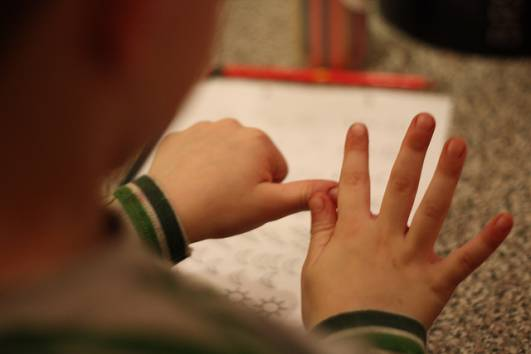# What is 9/20, in percent form?

In percent form, 9/20 is 45% (which can also be written as 45/100).

How To Convert 9/20 Into A Percentage

To convert a fraction into a percentage, you need to make the bottom number equal to 100, and then multiply the top number by however many times you multiplied the bottom number by.

• 9/20 is an easy fraction to convert, as 100 = 20 x 5.
• As you've multiplied the bottom number by 5, you must then do the same to the top number, which gives you 9 x 5 = 45.
• This leaves you with 45/100, which is equal to 45%.thanked the writer.